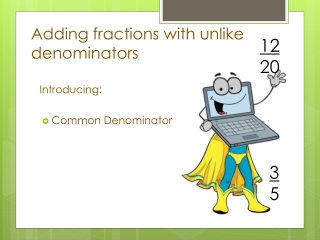DownloadDownload Presentation# Adding fractions with unlike denominators

Télécharger la présentation## Adding fractions with unlike denominators

- - - - - - - - - - - - - - - - - - - - - - - - - - - E N D - - - - - - - - - - - - - - - - - - - - - - - - - - -
##### Presentation Transcript

1. 1220 Adding fractions with unlike denominators Introducing: • Common Denominator 35

2. You know how to add problems with the same denominator, like this 35 45 15 = +

3. But what about problems like this? 35 ?? 14 = +

4. Before you can add or subtract fractions … You need to find a common denominator

5. HOW do you find the common denominator? No, you don’t find it by looking under a bush! Stop that!

6. A common denominator is When fractions have the SAME denominator, that is, they have the denominator in common

7. To find common denominator Would you stop that!

8. To find common denominator You need to find a number that is a multiple of each of the denominators.

9. To find common denominator Another way of saying that is that you need to find a number that can be divided by both of the denominators

10. AN EXAMPLE 35 • 1220 34 1520 The common denominator of 5 and 4 is 20 because both 5 and 4 divide evenly into 20.

11. AN EXAMPLE 35 • 1220 44 x 34 1520 Multiply the NUMERATOR AND DENOMINATOR by the same number to get an equivalent fraction

12. AN EXAMPLE 35 • 1220 34 55 1520 x Multiply the NUMERATOR AND DENOMINATOR by the same number to get an equivalent fraction

13. It’s called an EQUIVALENT fraction because it’s equal (another word for equivalent). It’s the SAME. 34 55 1520 x

14. It’s called an EQUIVALENT fraction because it’s equal (another word for equivalent). It’s the SAME. 34 55 1520 x 34 1520 Whether we call it or it’s still one and the same fraction

15. Don’t let this trip you up!

16. 35 • 1220 44 x 34 55 1520 x The two different fractions are multiplied by different numbers.

17. Because 5 x 4 = 20But 4 x 5 = 20 35 • 1220 44 x 34 55 1520 x

18. Think about it for a minute. No, seriously. Think about it. I’ll wait.

19. Common Denominators: An example

20. Koko, Misu, and Tama decided to cross the lake. Koko rows of the trip while Misu rows of the trip. 12 25

21. Koko, Misu, and Tama decided to cross the lake. Koko rows of the trip while Misu rows of the trip. 12 25 What fraction of the lake have they rowed?

22. 25 22 410 x = 12 55 510 x =

23. 510 410 910 + = LAZY !

24. To find a common denominator: 34 Think of the denominators 4 and 8 in and . Does the smaller denominator 4 divide evenly into the larger 8? Yes, then the larger denominator 8 is the common denominator. 58

25. If the smaller denominator does not divide evenly into the larger, multiply the larger denominator by 2, 3, and then 4, and so on. Each time check for division by the smaller denominator.